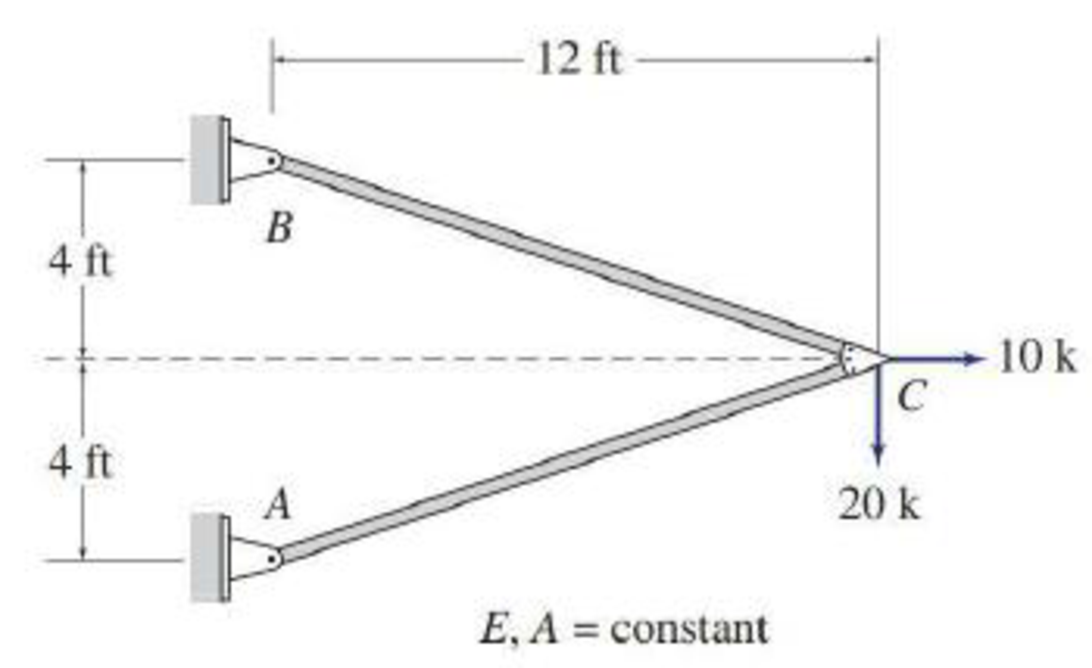# Determine the force in each member of the trusses shown in Figs. P10.1–P10.5 by utilizing structural symmetry. FIG. P10.2, P10.17

#### Solutions

Chapter
Section
Chapter 10, Problem 17P
Textbook Problem
1 views

## Determine the force in each member of the trusses shown in Figs. P10.1–P10.5 by utilizing structural symmetry.FIG. P10.2, P10.17

To determine

Find the force in each member of the truss using structural symmetry.

### Explanation of Solution

Given information:

The structure is given in the Figure.

The young’s modulus E and area A is constant.

Apply the sign conventions for calculating reactions, forces, and moments using the three equations of equilibrium as shown below.

• For summation of forces along x-direction is equal to zero (Fx=0), consider the forces acting towards right side as positive (+) and the forces acting towards left side as negative ().
• For summation of forces along y-direction is equal to zero (Fy=0), consider the upward force as positive (+) and the downward force as negative ().
• For summation of moment about a point is equal to zero (Matapoint=0), consider the clockwise moment as negative and the counter clockwise moment as positive.

Method of joints:

The negative value of force in any member indicates compression (C) and the positive value of force in any member indicates tension (T).

Calculation:

Refer the given structure.

The structure is symmetric with respect to the s axis.

Sketch the half loading for the given structure as shown in Figure 1.

Sketch the substructure with symmetric boundary conditions as shown in Figure 5.

Find the reactions and member end forces of substructure using equilibrium equations and to the left of s axis.

The member end forces to the right of s axis are obtained by the reflection.

Summation of moments about B is equal to 0.

MB=05×4Cy×12=0Cy=1.67k

Summation of forces along y-direction is equal to 0.

+Fy=0ByCy=0By1.67=0By=1.67k

Summation of forces along x-direction is equal to 0.

+Fx=05Bx=0Bx=5k

Consider joint C, find the force in the member BC:

Find the angle θ made by the member BC with respect to the horizontal axis using the given Figure

### Still sussing out bartleby?

Check out a sample textbook solution.

See a sample solution

#### The Solution to Your Study Problems

Bartleby provides explanations to thousands of textbook problems written by our experts, many with advanced degrees!

Get Started

Find more solutions based on key concepts
Solve Problem 20.3 using Excel.

Engineering Fundamentals: An Introduction to Engineering (MindTap Course List)

What are CSS, and are how arc they used?

Fundamentals of Information Systems

Which of the systems are equivalent to the couple in (a)?

International Edition---engineering Mechanics: Statics, 4th Edition

command language a. operating system problem that occurs when it spends much of its time paging, instead of exe...

Enhanced Discovering Computers 2017 (Shelly Cashman Series) (MindTap Course List)

Create the table that would result from stu_code, dept_code (student professor).

Database Systems: Design, Implementation, & Management

List and discuss some of the factors that influenced the evolution of the DDBMS.

Database Systems: Design, Implementation, & Management

If your motherboard supports ECC DDR3 memory, can you substitute non-ECC DDR3 memory?

A+ Guide to Hardware (Standalone Book) (MindTap Course List)